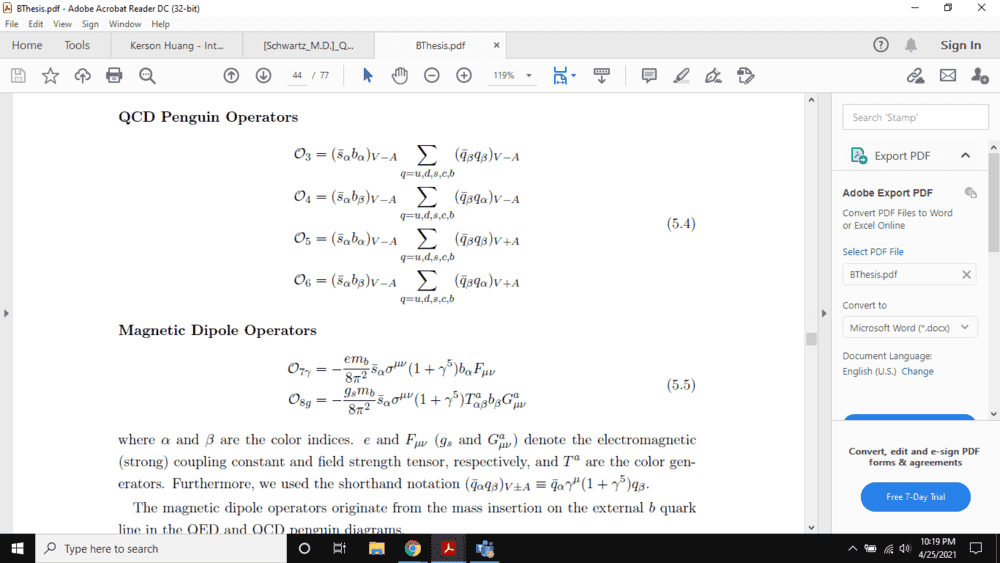# The vertex factors in QCD penguin operators

• A
Summary:
Not sure why there is a (V+A) term in here.
Have a look at O5 & O6 in Eqtns(5.4) . Why is there a (V+A) ?
(V+A) contains the projection operator which projects out the right Weyl from a Dirac spinor.
As per the Feynman rules of electroweak theory, there is a (V-A) assigned to each (Dirac) spinor-W boson vertex because W only couple to left Weyl spinors.
In the corresponding penguin diagrams there is the quark-antiquark loop coupling to the W so shouldn't both vertices have the same (V-A) factor always ?Staff Emeritus
Summary:: Not sure why there is a (V+A) term in here.

so shouldn't both vertices have the same (V-A) factor always ?

Maybe. You didn't post enough for us to see what is being calculated. We therefore can't tell what should or should not be there.

•Elmo
Maybe. You didn't post enough for us to see what is being calculated. We therefore can't tell what should or should not be there.
oh sorry.np Here it is.

Staff Emeritus